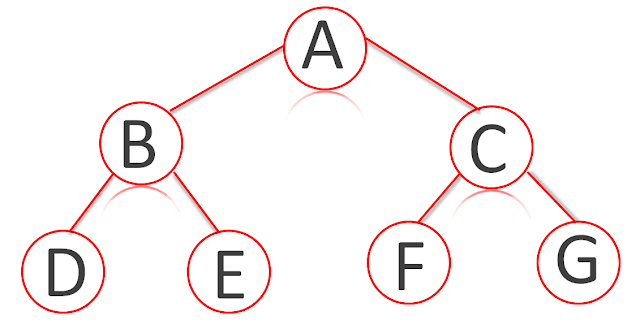# What is Tree Data Structure - Basic Terminologies Related to Trees

Tags: node tree nodesA Tree is a non-linear data structure. It is a set of nodes connected by edges. Each Node is a data structure consisting of a value together with a list of nodes (the "children"). In a tree, no node is duplicated. In a linked list, each node has a link which points to next node (as well as points to previous node in case of doubly linked list). In a tree structure, however, each node may point to several other nodes.

The tree structure is used to store data in a hierarchical manner. Generally, this data structure is used to organize the data so that items of information are related by branches. An example of a tree is a company’s organizational chart.

A tree starts with a root node and extends into several nodes. The root node is the first node in a tree and it may contain several links. Each link in the root node refers to a child. Thus, a tree is very powerful data structure that can be used in various applications.

In computer applications, tree is a hierarchical collection of finite elements. Each element is called a node. A typical tree is shown in the following diagram:## Basic Terminologies Related to Trees

Following are the basic terminologies, with their brief description.

### Root Node

The top most node in the tree that has no parent node is called the root node. It represents the beginning of the tree. Element A in the above figure is the root node of the tree.

### Parent Node

A node that has one child or more children is called parent node. It is also known as ancestor node, or superior. It is always above its child nodes. The node A in the above figure is the root. It is also the parent node of nodes B, C, and D. Similarly, node B is parent node of nodes E and F, node C is parent node of node G, node D is parent node of node H and so on.

### Child Node

The node that is directly connected to a parent node is called the child node. In the above diagram, nodes B, C, and D are the Child nodes of A.

### Subtree

The child node of 'the root" node that has its own child nodes is called the subtree. The nodes B, C, D, E, G and H in the above diagram are subtrees and are roots of their subtrees.

### Terminal Node

The node having no successor or child is called the terminal node. It is also called leaf or leaf node. The nodes, F, J, M, N, and L in the above diagram are terminal nodes or leaves.

### Level of a Node

The root of the tree has level 0 and level of any other node, in the tree is one more than the level of its parent. If a node is at level ‘i’ then its children are at level ‘i+1’. For example in the tree of the above diagram, the nodes I, J, K, and L are at level 3 and M,& N are at level 4.

### Siblings

The nodes having a common parent are called siblings. These are children of the same parent node. The nodes E and F in the above diagram are siblings as they are children of node B.

### Depth of Node

Each node has a unique path connecting it tothe root. The total number of nodes between the path of a specific node and the root is called depth of node. The depth of root node is zero.

### Height of Tree

The maximum depth among all of the nodes of a tree is called the height of the tree. The depth of each node M & N in the above diagram is 4. It is the maximum height of any node in the tree.

### Empty Tree

A tree that has no node is called empty tree. Its height is 1.

### Degree of Node

The number of children of a node is called degree of the node. In the above diagram, the degree of the node B is one and the degree of node K is two.

### Singleton Tree

The tree whose root is its only node is called singleton tree.

### General Tree

A tree in which a node may have no child, one child or any number of children is called general tree.

### Full Tree

A tree whose all-internal nodes have the same degree and all its terminals are at the same level is called full tree. The tree in the following image is an example of a full tree.Read: Important File Operation Modes in C++

This post first appeared on Programming Explain, please read the originial post: here

# Share the post

What is Tree Data Structure - Basic Terminologies Related to Trees

×